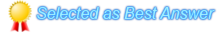You must Sign In to post a response.
• # How to write linq query in mvc using lambda expression

hi all i have three tables ordrrsptrk,po-ms and po -li -ams so what i want is i want select data from these three tables using linq query and satisfying conditions like ordrrsptrk.orderid=poams.orderid and pan-ordrrsptrk.ordernum = poams.oda001 and polineams.odb001=ordrsptrk.linenum and polineams.odboo2=ordrsptrk.buyerpartno
•Hi,

We can easily achieve this using basic queries using Lambda Expressions,
Before going into those if we know the clear expression like how to call those keywords or condition we can easily achieve it.

How to call the Where condition?
`IEnumerable<Order> x = order.Where(p => p.UnitPrice >= 10); IEnumerable<Order> x = from o in order where o.UnitPrice >= 10 select o;`

Now Select query,
`IEnumerable<string> orderDetails= order.Select(p => p.Name);(or) IEnumerable<string> orderDetails= from p in order select p.Name;var namesAndPrices = order. Where(p => p.UnitPrice >= 10). Select(p => new { p.Name, p.UnitPrice }). ToList();IEnumerable<int> indices = order. Select((order, index) => new { order, index }). Where(x => x.order.UnitPrice >= 10). Select(x => x.index);`

For your requirement, I feel you need Join conditions. So we can use join like following ways in LINQ
`var custOrders =customers. Join(orders, c => c.CustomerID, o => o.CustomerID, (c, o) => new { c.Name, o.OrderDate, o.Total } );var custTotalOrders = customers. GroupJoin(orders, c => c.CustomerID, o => o.CustomerID, (c, co) => new { c.Name, TotalOrders = co.Sum(o => o.Total) } );`

Thanks,
Mani

•The Lambda expressions are expressed by the following syntax:
(input parameters) => expression or statement block
Example of lambda expression
y => y * y
Example code snippet for use of lambda expression`namespace lambdaexample{ class Program { delegate int del(int i); static void Main(string[] args) { del myDelegate = y => y * y; int j = myDelegate(5); Console.WriteLine(j); Console.ReadLine(); } }}`
Useful reference: https://msdn.microsoft.com/en-us/library/bb397675.aspx